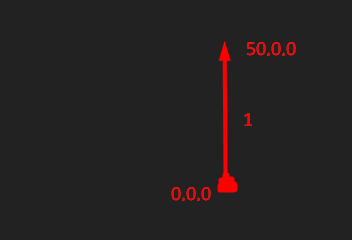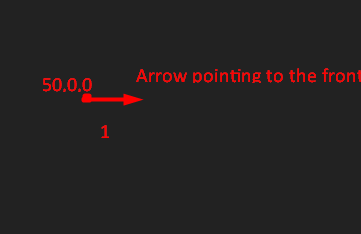# Need help understanding .unit and look vector

I just watched a dev king tutorial on Raycasting, I am having trouble understanding the following lines of code, can someone please help me understand them, thank you.

(Line 20)

(Line 34)

4 Likes

`LookVector` is the direction the `CFrame` is facing in, and `Unit` is just a unit measurement(so everything is compressed into a ratio of 1).

4 Likes

But why are we multiplying look vector with speed?

1 Like

Oh! NVM I understand now, thank you. Can you please explain unit in more detail and what its advantages are?

2 Likes

`Unit` is good because it gives you a single vector (or arrow) that has a length (length is also called `magnitude` of 1. So, for example if the numbers were `1, 2, 3`, the unit of that would be `0.267, 0.535, 0.802`. This is because the length of that is 1.

``````print(Vector3.new(0.267, 0.535, 0.802).Magnitude) -- around 1
``````
4 Likes

.Unit returns a type of "“arrow” pointing to a position and its length is 1, look vector is a unit that points towards where the object is looking at.

So as a example: Doing Vector3.new(50,0,0).Unit will return a arrow like thisThe 1 is the length of the arrow. So now using look vector as a example:

Part.CFrame.LookVector (And the CFrame of the position is at 50,0,0)3 Likes

So is using unit a necessity or does it help getting better results?

1 Like

Unit in this context is used so the ray is always 500 studs long because Unit returns a vector with the same direction but with the length of 1. If you don’t use Unit, the resulting ray’s length is unknown and can eventually be much shorter than you intended and not function correctly.

4 Likes

Its the only way for this to work for the most part. You need to fire the ray in a direction, and a unit vector represents a direction and magnitude (basically distance in that direction) of 1.

2 Likes

Unit is a necessity when you are using things like rays since it requires the direction. (Again, LookVector is also a Unit so you can use it as a ray direction)

Edit: In the case of rays you need the unit and multiply it by the length you want it to be. So you will have an arrow pointing to a place and with a length (So you can detect the things inside that arrow with a certain extent)

4 Likes

@BanTech can you please explain .Unit? It’s super confusing.

1 Like

This should do.

If you need to know why Unit is used, refer to my previous comment.

5 Likes

The `Unit` property normalizes the vector. It just makes the length of your vector to “1” (the unit number) but preserves the angle. Multiplying a new scalar to it will change the length of the vector.

`Ray.new(turret.Position, (torso.Position - turret.Position).Unit * 500)` creates a ray from `turret.Position` towards the normalized vector for `(torso.Position - turret.Position)` multiplied by 500. Essentially just go from `turrent.Position` to 500 studs in the torso’s direction.

3 Likes

I think what they’re trying to explain is this.
if you move a part 30 studs away the max length moved would be 30 so in units 30 would be 1 if you divide 30 by 2 it would be 15 so in units it would be 0.5, from what I’ve understood from the topics above .Unit is basically a way to measure length starting from 0 to 1 and between 0 to 1 there’s like 0.1, 0.01, (0.01 is the minimum length and 1 is the maximum I think).

1 Like

Please stop tagging me in each support topic you create. Other members of the forum can generally explain just as well.

Unit simply normalising the length of the vector to a length of 1.

If you had a vector (0, 3, 4) it would have a length of 5. Its normalised vector is therefore (0, 0.6, 0.8). It’s the same as dividing the vector by its magnitude.

`Vector.Unit = Vector / Vector.Magnitude`

2 Likes

Thank you, I am really sorry for tagging you in my support topics, I will not do it from now on. Again I am deeply sorry.

1 Like

Hey! Thank you so much for your explanation, my question is how does converting it to the length of 1 help? I mean shouldn’t multiplying it by 500 work regardless?

1 Like

Judging from the code you provided I can’t say for sure, but it looks like it should work fine regardless if you used Unit or not.
There are cases (for example raycast guns, where you would specify its “range” with the length of its casted ray) where you need a constant ray length and if you don’t know how long your direction vector is, you multiply the unit vector with the desired length.

1 Like

Is there a Roblox Developer page article on .Unit?

1 Like

So, if I multiply 500 to the vector without using .Unit it will not work properly, however if I use .Unit it will?

1 Like